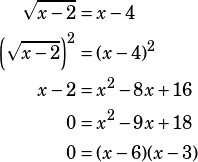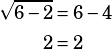College Board Test 4 Section 3 #9:
____
√x-a = x-4
If a=2 what is the solution set of the preceding equation?
A. {3, 6}
B. {2}
C. {3}
D. {6}

Is there another way to solve this quickly besides plugging in the answer choices?

Backsolve is far and away my preferred method on a question like this. The answer choices only show you 2, 3, and 6 as possible solutions, so you have to try, at most, three numbers.

However, of course there is an algebraic solution! If you go that way, you must be careful because you will generate extraneous solutions. To begin, square both sides, combine like terms, and solve the resulting quadratic:From there, you must check that both solutions you generated actually work in the original equation, because when you square both sides of an equation you can cause extraneous solutions.As you can see, 3 doesn’t work in the original equation, so it’s an extraneous solution. 6 DOES work in the original equation, so it’s the one we go with.

Hopefully this reinforces the appeal of backsolving here: even if you do the algebra, you still need to try the answers you find in the original equation anyway. Why not just start by trying the answers provided!? 🙂